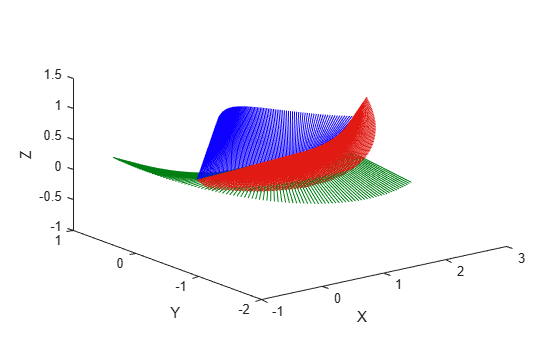# transformtraj

Generate trajectories between two transformations

## Syntax

``[tforms,vel,acc] = transformtraj(T0,TF,tInterval,tSamples)``
``[tforms,vel,acc] = transformtraj(T0,TF,tInterval,tSamples,Name,Value)``

## Description

example

````[tforms,vel,acc] = transformtraj(T0,TF,tInterval,tSamples)` generates a trajectory that interpolates between two 4-by-4 homogeneous transformations, `T0` and `TF`, with points based on the time interval and given time samples.```
````[tforms,vel,acc] = transformtraj(T0,TF,tInterval,tSamples,Name,Value)` specifies additional parameters using `Name,Value` pair arguments.```

## Examples

collapse all

Build transformations from two orientations and positions. Specifiy the time interval and vector of times for interpolating.

```t0 = axang2tform([0 1 1 pi/4])*trvec2tform([0 0 0]); tF = axang2tform([1 0 1 6*pi/5])*trvec2tform([1 1 1]); tInterval = [0 1]; tvec = 0:0.01:1;```

Interpolate between the points. Plot the trajectory using `plotTransforms`. Convert the transformations to quaternion rotations and linear transitions. The figure shows all the intermediate transformations of the coordinate frame.

```[tfInterp, v1, a1] = transformtraj(t0,tF,tInterval,tvec); rotations = tform2quat(tfInterp); translations = tform2trvec(tfInterp); plotTransforms(translations,rotations) xlabel('X') ylabel('Y') zlabel('Z')```## Input Arguments

collapse all

Initial transformation, specified as a 4-by-4 homogeneous transformation. The function generates a trajectory that starts at the initial transformation, `T0`, and goes to the final transformation, `TF`.

Data Types: `single` | `double`

Final transformation, specified as a 4-by-4 homogeneous transformation. The function generates a trajectory that starts at the initial transformation, `T0`, and goes to the final transformation, `TF`.

Data Types: `single` | `double`

Start and end times for the trajectory, specified as a two-element vector in seconds.

Example: `[0 10]`

Data Types: `single` | `double`

Time samples for the trajectory, specified as an m-element vector in seconds. The output trajectory, `rotVector`, is a vector of orientations.

Example: `0:0.01:10`

Data Types: `single` | `double`

### Name-Value Pair Arguments

Specify optional comma-separated pairs of `Name,Value` arguments. `Name` is the argument name and `Value` is the corresponding value. `Name` must appear inside quotes. You can specify several name and value pair arguments in any order as `Name1,Value1,...,NameN,ValueN`.

Example: `'TimeScaling',[0 1 2; 0 1 0; 0 0 0]`

Time scaling vector and the first two derivatives, specified as a 3-by-m vector, where m is the length of `tSamples`. By default, the time scaling is a linear time scaling between the time points in `tInterval`.

For a nonlinear time scaling, specify the values of the time points as positions in meters in the first row. The second and third rows are the velocity and acceleration of the time points in m/s and m/s2, respectively. For example, to follow the path with a linear velocity to the halfway point, and then jump to the end, the time-scaling would be:

```s(1,:) = [0 0.25 0.5 1 1 1] % Position s(2,:) = [1 1 1 0 0 0] % Velocity s(3,:) = [0 0 0 0 0 0] % Acceleration```

Data Types: `single` | `double`

## Output Arguments

collapse all

Transformation trajectory, returned as a 4-by-4-by-m homogeneous transformation matrix array, where m is the number of points in `tSamples`.

Transformation velocities, returned as a 6-by-m matrix in m/s, where m is the number of points in `tSamples`. The first three elements are the angular velocities, and the second three elements are the velocities in time.

Transformation accelerations, returned as a 6-by-m matrix in m/s2, where m is the number of points in `tSamples`. The first three elements are the angular accelerations, and the second three elements are the accelerations in time.